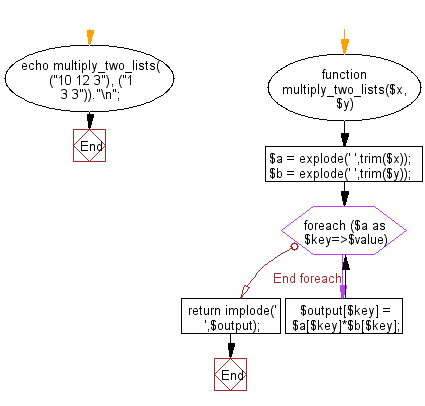﻿ PHP Exercise: Multiplies corresponding elements of two given lists - w3resource# PHP Exercises: Multiplies corresponding elements of two given lists

## PHP: Exercise-43 with Solution

Write a PHP program that multiplies corresponding elements of two given lists.

Sample Solution: -

PHP Code:

``````<?php
function multiply_two_lists(\$x, \$y)
{
\$a = explode(' ',trim(\$x));
\$b = explode(' ',trim(\$y));
foreach(\$a as \$key=>\$value){
\$output[\$key] = \$a[\$key]*\$b[\$key];
}
return implode(' ',\$output);
}
echo multiply_two_lists(("10 12 3"), ("1 3 3"))."\n";
?>
```
```

Sample Output:

```10 36 9
```

Flowchart:PHP Code Editor:

Have another way to solve this solution? Contribute your code (and comments) through Disqus.

What is the difficulty level of this exercise?

﻿

## PHP: Tips of the Day

Returning JSON from a PHP Script

While you're usually fine without it, you can and should set the Content-Type header:

Example:

```<?PHP
\$data = /** whatever you're serializing **/;